# Arrays

21. Jan 2022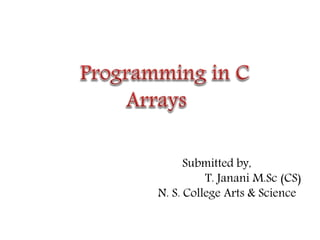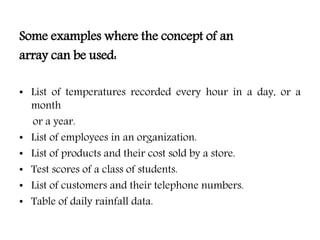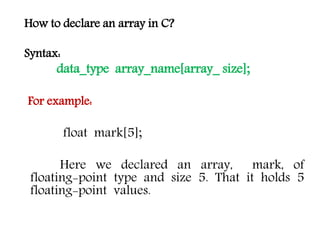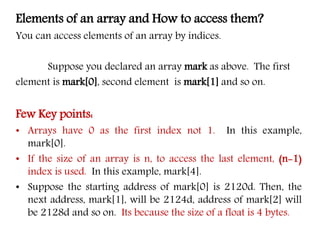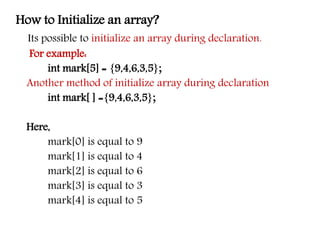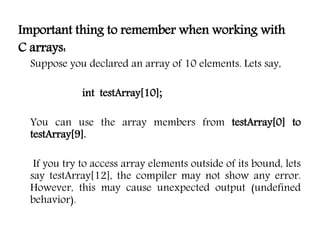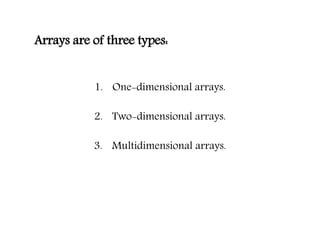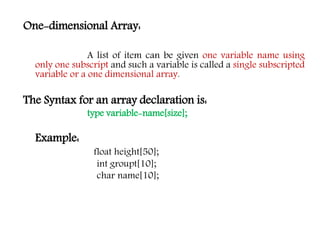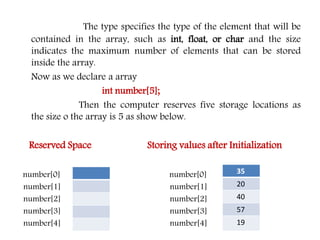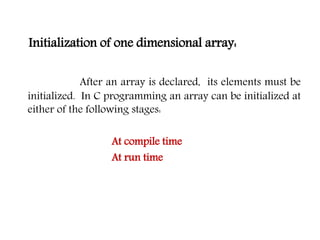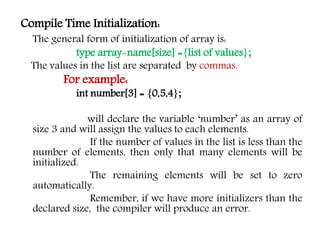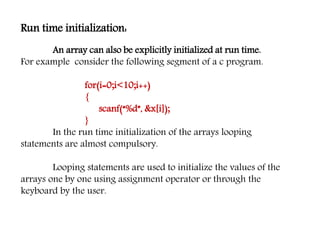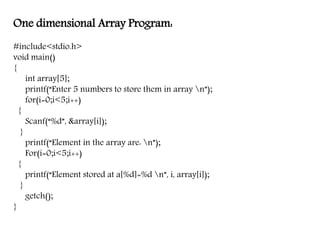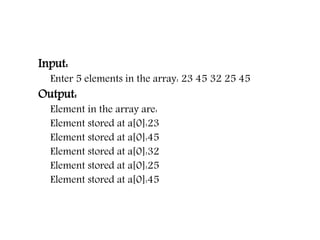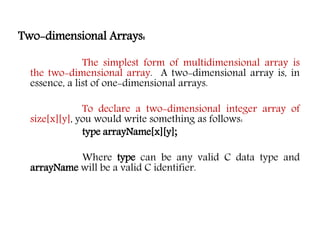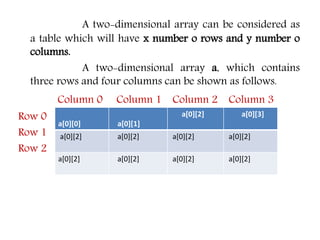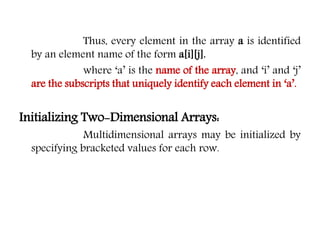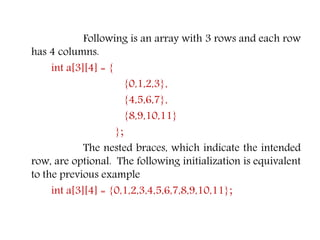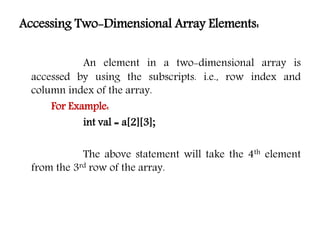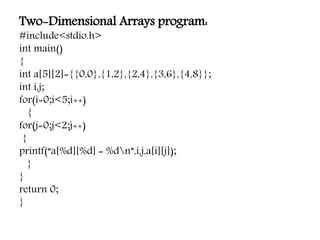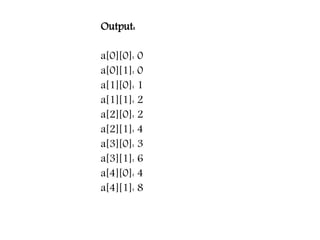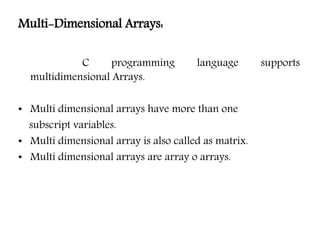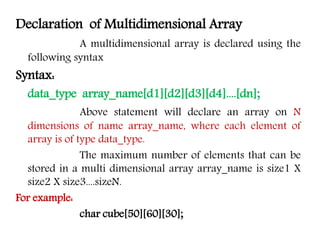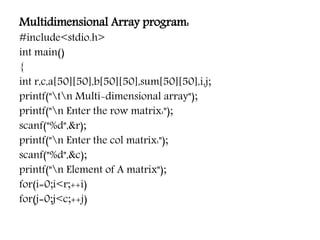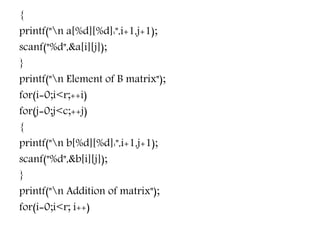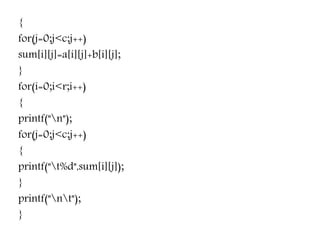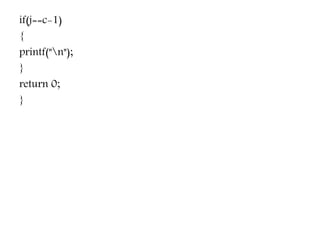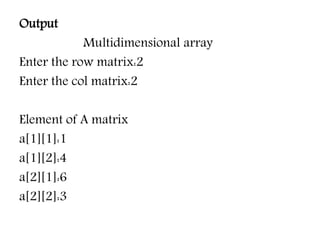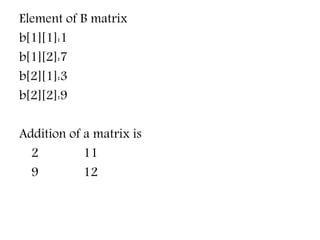1 von 31

### Arrays

• 1. Submitted by, T. Janani M.Sc (CS) N. S. College Arts & Science
• 2. Arrays: An array is a collection of data that holds fixed number of values of same type. The size and type of arrays cannot be changed after its declaration. For Example : If you want to store marks of 100 students you can create an array for it. float marks;
• 3. Some examples where the concept of an array can be used: • List of temperatures recorded every hour in a day, or a month or a year. • List of employees in an organization. • List of products and their cost sold by a store. • Test scores of a class of students. • List of customers and their telephone numbers. • Table of daily rainfall data.
• 4. How to declare an array in C? Syntax: data_type array_name[array_ size]; For example: float mark; Here we declared an array, mark, of floating-point type and size 5. That it holds 5 floating-point values.
• 5. Elements of an array and How to access them? You can access elements of an array by indices. Suppose you declared an array mark as above. The first element is mark, second element is mark and so on. Few Key points: • Arrays have 0 as the first index not 1. In this example, mark. • If the size of an array is n, to access the last element, (n-1) index is used. In this example, mark. • Suppose the starting address of mark is 2120d. Then, the next address, mark, will be 2124d, address of mark will be 2128d and so on. Its because the size of a float is 4 bytes.
• 6. How to Initialize an array? Its possible to initialize an array during declaration. For example: int mark = {9,4,6,3,5}; Another method of initialize array during declaration int mark[ ] ={9,4,6,3,5}; Here, mark is equal to 9 mark is equal to 4 mark is equal to 6 mark is equal to 3 mark is equal to 5
• 7. Important thing to remember when working with C arrays: Suppose you declared an array of 10 elements. Lets say, int testArray; You can use the array members from testArray to testArray. If you try to access array elements outside of its bound, lets say testArray, the compiler may not show any error. However, this may cause unexpected output (undefined behavior).
• 8. Arrays are of three types: 1. One-dimensional arrays. 2. Two-dimensional arrays. 3. Multidimensional arrays.
• 9. One-dimensional Array: A list of item can be given one variable name using only one subscript and such a variable is called a single subscripted variable or a one dimensional array. The Syntax for an array declaration is: type variable-name[size]; Example: float height; int groupt; char name;
• 10. The type specifies the type of the element that will be contained in the array, such as int, float, or char and the size indicates the maximum number of elements that can be stored inside the array. Now as we declare a array int number; Then the computer reserves five storage locations as the size o the array is 5 as show below. Reserved Space Storing values after Initialization number number number number number number number number number number 35 20 40 57 19
• 11. Initialization of one dimensional array: After an array is declared, its elements must be initialized. In C programming an array can be initialized at either of the following stages: At compile time At run time
• 12. Compile Time Initialization: The general form of initialization of array is: type array-name[size] ={list of values}; The values in the list are separated by commas. For example: int number = {0,5,4}; will declare the variable ‘number’ as an array of size 3 and will assign the values to each elements. If the number of values in the list is less than the number of elements, then only that many elements will be initialized. The remaining elements will be set to zero automatically. Remember, if we have more initializers than the declared size, the compiler will produce an error.
• 13. Run time initialization: An array can also be explicitly initialized at run time. For example consider the following segment of a c program. for(i=0;i<10;i++) { scanf(“%d”, &x[i]); } In the run time initialization of the arrays looping statements are almost compulsory. Looping statements are used to initialize the values of the arrays one by one using assignment operator or through the keyboard by the user.
• 14. One dimensional Array Program: #include<stdio.h> void main() { int array; printf(“Enter 5 numbers to store them in array n”); for(i=0;i<5;i++) { Scanf(“%d”, &array[i]); } printf(“Element in the array are: n”); For(i=0;i<5;i++) { printf(“Element stored at a[%d]=%d n”, i, array[i]); } getch(); }
• 15. Input: Enter 5 elements in the array: 23 45 32 25 45 Output: Element in the array are: Element stored at a:23 Element stored at a:45 Element stored at a:32 Element stored at a:25 Element stored at a:45
• 16. Two-dimensional Arrays: The simplest form of multidimensional array is the two-dimensional array. A two-dimensional array is, in essence, a list of one-dimensional arrays. To declare a two-dimensional integer array of size[x][y], you would write something as follows: type arrayName[x][y]; Where type can be any valid C data type and arrayName will be a valid C identifier.
• 17. A two-dimensional array can be considered as a table which will have x number o rows and y number o columns. A two-dimensional array a, which contains three rows and four columns can be shown as follows. Column 0 Column 1 Column 2 Column 3 Row 0 Row 1 Row 2 a a a a a a a a a a a a
• 18. Thus, every element in the array a is identified by an element name of the form a[i][j]. where ‘a’ is the name of the array, and ‘i’ and ‘j’ are the subscripts that uniquely identify each element in ‘a’. Initializing Two-Dimensional Arrays: Multidimensional arrays may be initialized by specifying bracketed values for each row.
• 19. Following is an array with 3 rows and each row has 4 columns. int a = { {0,1,2,3}, {4,5,6,7}, {8,9,10,11} }; The nested braces, which indicate the intended row, are optional. The following initialization is equivalent to the previous example int a = {0,1,2,3,4,5,6,7,8,9,10,11};
• 20. Accessing Two-Dimensional Array Elements: An element in a two-dimensional array is accessed by using the subscripts. i.e., row index and column index of the array. For Example: int val = a; The above statement will take the 4th element from the 3rd row of the array.
• 21. Two-Dimensional Arrays program: #include<stdio.h> int main() { int a={{0,0},{1,2},{2,4},{3,6},{4,8}}; int i,j; for(i=0;i<5;i++) { for(j=0;j<2;j++) { printf(“a[%d][%d] = %dn”,i,j,a[i][j]); } } return 0; }
• 22. Output: a: 0 a: 0 a: 1 a: 2 a: 2 a: 4 a: 3 a: 6 a: 4 a: 8
• 23. Multi-Dimensional Arrays: C programming language supports multidimensional Arrays. • Multi dimensional arrays have more than one subscript variables. • Multi dimensional array is also called as matrix. • Multi dimensional arrays are array o arrays.
• 24. Declaration of Multidimensional Array A multidimensional array is declared using the following syntax Syntax: data_type array_name[d1][d2][d3][d4]....[dn]; Above statement will declare an array on N dimensions of name array_name, where each element of array is of type data_type. The maximum number of elements that can be stored in a multi dimensional array array_name is size1 X size2 X size3....sizeN. For example: char cube;
• 25. Multidimensional Array program: #include<stdio.h> int main() { int r,c,a,b,sum,i,j; printf("tn Multi-dimensional array"); printf("n Enter the row matrix:"); scanf("%d",&r); printf("n Enter the col matrix:"); scanf("%d",&c); printf("n Element of A matrix"); for(i=0;i<r;++i) for(j=0;j<c;++j)
• 26. { printf("n a[%d][%d]:",i+1,j+1); scanf("%d",&a[i][j]); } printf("n Element of B matrix"); for(i=0;i<r;++i) for(j=0;j<c;++j) { printf("n b[%d][%d]:",i+1,j+1); scanf("%d",&b[i][j]); } printf("n Addition of matrix"); for(i=0;i<r; i++)
• 29. Output Multidimensional array Enter the row matrix:2 Enter the col matrix:2 Element of A matrix a:1 a:4 a:6 a:3
• 30. Element of B matrix b:1 b:7 b:3 b:9 Addition of a matrix is 2 11 9 12